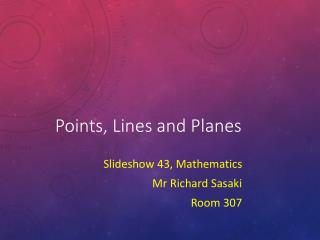DownloadDownload PresentationPoints, Lines and Planes

# Points, Lines and Planes

Download Presentation## Points, Lines and Planes

- - - - - - - - - - - - - - - - - - - - - - - - - - - E N D - - - - - - - - - - - - - - - - - - - - - - - - - - -
##### Presentation Transcript

1. Points, Lines and Planes Slideshow 43, Mathematics Mr Richard Sasaki Room 307

2. Objectives Recall names of some common 3d Shapes Understand the meaning of a point, line and plane and notation used Use these properties to answer problems

3. Some Simple 3-D Shapes Cylinder Cone Sphere Square-based pyramid Hemisphere

4. Platonic solids / concave regular polyhedrons Cube Tetrahedron Octahedron Dodecahedron Icosahedron

5. Prisms Triangular Prism Cuboid Pentagonal Prism Hexagonal Prism Octagonal Prism Decagonal Prism

6. Points A point is represented by a dot and its name. It has zero size in all directions. Position only. So what does it have? A What is a point? (A point is different to a vertex…any ideas how?) A vertex is usually used to connect lines and line segments. A point may be separate. A vertex is a type of point.

7. Lines We already know this. It is infinite in length and travels in opposite directions about its centre. What is a line? As we’re not always interested in vertices, we can just simply name a line at times.

8. Planes No it doesn’t fly in the sky! A plane is a flat 2D surface. It is often thought of to be infinite in length. What is a plane? As with lines, we can simply name them but they may be named about their vertices if stated (eg: ABCD).

9. Intersection We call the line formed between them the . line of intersection (The planes don’t have to be perpendicular to intersect, remember!) Look at the two intersecting planes below.

10. Parallel elements No! They must always be the same distance apart at all points. No! They must always be the same distance apart at all points. In the land of 3D, any two pairs of lines that never touch must be parallel. Is this true? We call lines that are not parallel and never touch . skew lines

11. Parallel elements Are these lines parallel (by appearance)? How about these planes? Lines and planes can also be parallel as long as a parallel line of points on the plane are always equal distance to the physical line. For this figure, as an example.

12. 1 – Line of intersection Answers - Easy Yes, the plane must be flat so the flat line will lie on it. Face DCFE A circle. (Opposite order of letters is okay.) A train track/ ladder / fence.

13. The sphere only has one curved face. A straight line or flat plane will have differing distances from the sphere’s surface. Answers - Hard 45o One No, because it has one flat surface. 2 2 2 2 No, the pencil must sit on the line but it can at any angle perpendicular to the line.